# The Ultimate Guide To Boolean Logic Operations & DeMorgan’s Theorems

## Boolean Logic Operations & DeMorgan’s Theorems

This is the ultimate guide to Boolean logic operations & DeMorgan’s Theorems.

### Boolean Logic Operations

A Boolean function is an algebraic expression formed using binary constants, binary variables and Boolean logic operations symbols.

Basic Boolean logic operations include the AND function (logical multiplication), the OR function (logical addition) and the NOT function (logical complementation).

A Boolean function can be converted into a logic diagram composed of the AND, OR and NOT gates.

#### Logical AND Operation:

The logical AND operation of two Boolean variables A and B, given as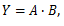The common symbol for this operation is the multiplication sign (.)

The Table-1 shows that the result of the AND operation on the variables A and B is logical 0 for all cases, except when both A and B are logical 1.

Usually, the dot denoting the AND function is omitted and (A . B) is written as AB.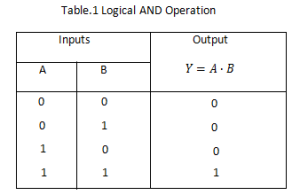#### Logical OR Operation:

The logical OR operation between two Boolean variables A and B, given as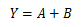The Table-2 shows that the result of the OR operation on the variables A and B is logical 1 when A or B  (or both) are logical 1.

The common symbol used for this logical addition operation is the plus sign (+).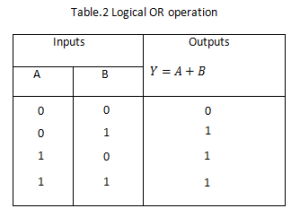#### Logical Complementation (Inversion):

The logical inverse operation converts the logical 1 to the logical 0 and vice versa.

This method is also called the NOT operation. The symbol used for this operation is a bar over the function or the variable.

Several notations, such as adding an asterisk, a star, prime, etc. over the variable, are used to indicate the NOT operation.

NOT A or the complement of A is represented by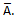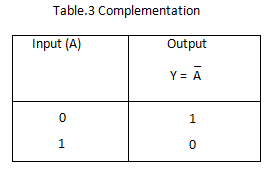#### Basic Laws of Boolean Algebra:

Logical operations can be expressed and minimized mathematically using the rules, laws, and theorems of Boolean algebra. It is a convenient and systematic method of expressing and analyzing the operation of digital circuits and systems.

Boolean algebra uses binary arithmetic variables which have two distinct symbols 0 and 1. These are called levels or states of logic. For example, a binary 1 represents a High level and a binary 0 represents a Low level.

Addition by the Boolean method involves variables having values of either a binary 1 or a 0. The basic rules of Boolean addition are given below: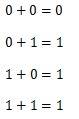Boolean addition is same as logical OR operation.

#### Boolean Multiplication:

The basic rules of the Boolean multiplication method are as follows:The Boolean multiplication is same as the logical AND operation.

#### Properties of Boolean Algebra:

Boolean algebra is a mathematical system consisting of a set of two or more distinct elements, two binary operators denoted by the symbols (+) and (.) and one unary operator denoted by the symbol either (-) or prime (’).

They satisfy the commutative, associative, distributive, absorption, consensus and idempotency properties of the Boolean algebra.

#### Commutative Property:

Boolean addition is commutative, given by:

According to this property, the order of the OR operation conducted on the variables make no difference.

Boolean algebra is also commutative over multiplication, given by:

This means that the order of the AND operation conducted on the variables makes no difference.

#### Associative Property:

The associative property of addition is given by:

The OR operation of several variables results in the same, regardless of the grouping of the variables.

The associative law of multiplication is given by:

According to this law, it makes no difference in what order the variables are grouped during the AND operation of several variables.

#### Distributive Property:

1. The Boolean addition is distributive over Boolean multiplication, given by:

This property states that the AND operation (multiplication) of several variables and then OR operation (addition) of the result with a single variable is equivalent to the OR operation of the single variable with each of the several variables and then the AND operation of the sums.

Proof: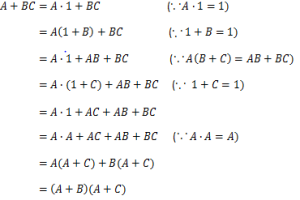2.   Boolean multiplication is also distributive over Boolean addition given by:

According to this property, the OR operation of several variables and then the AND operation of the result with a single variable is equivalent to the AND operation of the single variable with each of the several variables and then the OR operation of the products.

Absorption Laws:

Proof: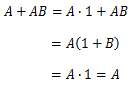Proof: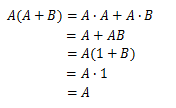Proof: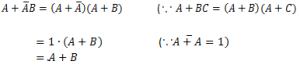Proof: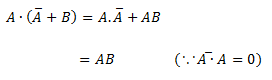Consensus laws:

Proof: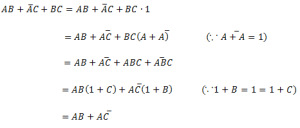Proof: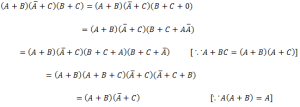The other basic laws of Boolean algebra are given below.  These theorems can be proved easily by adopting the truth table method or by using algebraic manipulation.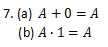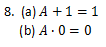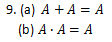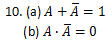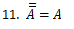Principle of Duality:

From the above properties and laws of Boolean algebra, it is evident that they are grouped in pairs as (a) and (b). One expression can be obtained from the other in each pair by replacing every 0 with 1, every 1 with 0, every (+) with (.), and every (.) with (+).

Any pair of expression satisfying this property is called dual expression. This characteristic of Boolean algebra is called the principle of duality.

### DEMORGAN’S Theorems

Two theorems that are an important part of Boolean algebra were proposed by DeMorgan.

The first theorem states that the complement of a product is equal to the sum of the complements. That is, if the variables are A and B, then,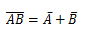The second theorem states that, the complement of a sum is equal to the product of the complements. That is: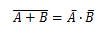The complement of a Boolean logic function or a logic expression may be expanded or simplified by following the steps of DeMorgan’s theorem.

• Replace the symbol (+) with symbol (.), the symbol (.) with symbol (+) given in the expression.
• Complement each of the terms or variables in the given expression.

DeMorgan’s theorem can be proved for any number of variables.

Proof of these theorems for 2-input variables is shown in Table-4.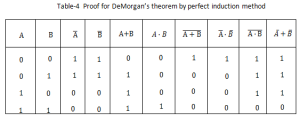A study of Table-4 makes clear that columns 7 and 8 are equal. Therefore,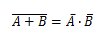Similarly, columns 9 and 10 are equal, therefore,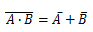DeMorgan’s theorem can also be proved by algebraic method as follows:

According to the first theorem,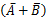, is the complement of  AB.

As we know from Boolean laws: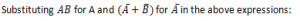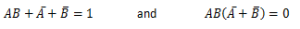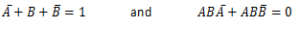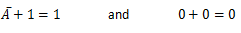Thus DeMorgan’s first theorem is proved algebraically.

Now substituting (A+B) for A and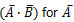, in the above Boolean expressions 10(a) and 10(b),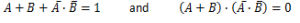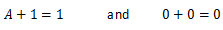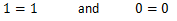Thus, DeMorgan’s second theorem is proved algebraically.